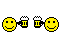1. ##Scripting - automating tasks in the V8 editor

The V8 editor supports the scripting language called Lua (https://www.lua.org/manual/5.3/contents.html#index).
The Lua scripts can be used to automate almost any task that you can do manually with the V8 editor.

You may automatically create a script based on the differences between two tune files using the [Create] button on the [F3: Tune]->[F9: Scripting] tab page - while you have two files open for comparison.
The created script can then be used to set the alternate file's calibrations to match the base file's calibrations.

All values that are read/written from/to the tune calibrations must be in metric units.
To convert between units use the _efiConvertValue function.

When comparing floating point values, rounding errors can cause comparisons for equality to fail when values are so close that they should be considered equal.
To compare floating point values EFILive recommends using the _efiCompareFlaot() function.

Example script to show how to use the _efiConvertValue() and the _efiCompareFloat() functions:

Code:
```val1=1.234
val2=1.2345
if ( _efiCompareFloat(val1,val2,0.0001) ) then
print("Same at 4 decimal places")
else
print("Diff at 4 decimal places")
end

if ( _efiCompareFloat(val1,val2,0.001) ) then
print("Same at 3 decimal places")
else
print("Diff at 3 decimal places")
end

kpa = 100
if ( psi==nil ) then
end

kpa = 100
psi = _efiConvertValue(kpa,"kPa","psi")
if ( psi==nil ) then
print(EFI_ErrMsg)
return
end

print(kpa.." kPa = "..psi.." psi");```
Output when this script is run:
Code:
```Diff at 4 decimal places
Same at 3 decimal places
Error: \$0806: tleNoConversion kpa -> badunitname
100 kPa = 14.50377 psi
Script returned: \$0000 (0)```Reply With Quote

2. ##A function for comparing float's is a very good idea( 0.1 * 10 != 1.0 )Reply With Quote

####Posting Permissions

• You may not post new threads
• You may not post replies
• You may not post attachments
• You may not edit your posts
•